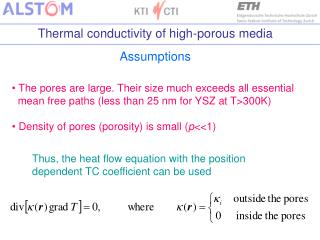DownloadDownload PresentationAssumptions

# Assumptions

Download Presentation## Assumptions

- - - - - - - - - - - - - - - - - - - - - - - - - - - E N D - - - - - - - - - - - - - - - - - - - - - - - - - - -
##### Presentation Transcript

1. Assumptions • The pores are large. Their size much exceeds all essential • mean free paths (less than 25 nm for YSZ at T>300K) • Density of pores (porosity) is small (p<<1) Thus, the heat flow equation with the position dependent TC coefficient can be used

2. heat flux T2 T1 L heat flux T1 T2 L TC of a homogeneous media: z Q - heat flux, S - cross-section Average TC of a non-homogeneous media: z

3. Approach TC is considered as a sum of its average and fluctuating components: is a random value. The results are evaluated via the average , which depends only on the distance between the pointsrand r’ For spherical pores, it is possible to assume: Fornon-spherical pores or cracks, it is possible to assume: determines ratio of the crack dimensions parameter Thus,≈ 1 for the pore, <<1 and>> 1 for the cracks of different orientations

4. Results Spherical pores Anisotropic pores (microcracks etc.)

5. Results (1) heat flux (= 1 - pore,  vary or small - crack) heat flux Equation (1) with J = 0.3 and p as a total porosity can be used to consider TC for all types of porosity

6. Comments • TC was found to be depended on the porosity, but independent of the pores size. This is the consequence of the model, which has not been assumed initially. This fact is confirmed experimentally . • The value of the factor J=1/3 for the spherical pores has been obtained also in Ref. 2 and confirmed experimentally . The close value J=1/2 has been tested in [1,3]. • The effect of anisotropy of TC along and across the plasma sprayed PSZ coating was found to be small in two different models of simulations  in agreement with Eq. (1). • References • K. Schlichting, N. Padture, P. Klemens. J. Materials Sci. 36, 3003 (2001) • K. Schlichting, N. Padture, P. Klemens, in “Thermal Conductivity 25” ed. By C. Uher and D. Morelli (Technomic, Lancaster, PA, USA, 2000) p.162 • S. Raghavan et al.,Scripta Materiala 39, 1119 (1998) • Z. Wang et al., Acta Materialia 51, 5319 (2003)

7. Unclear questions and Next steps • - Is it possible to consider porosity p = 0.3 as small? • Yes, because it results in correction ~p/3<<1 • No, the porosity p=0.7 means total destruction of the coating • - What value should be used as i ? • As the first approximation the intrinsic (defect-free) TC can be • used; nevertheless, the effect of the grain boundaries should be • testified. • Possible breaking bounds of the p<<1 approximation should be investigated • The radiative component of TC should be considered • Experimental data of TC measurements for the specimens of different porosity are necessary to check Eq. (1)